# 3D渲染中远处物体Z-Fighting(闪烁)问题

Z-Fighting就是深度冲突，当两个待渲染物体的三角形网格比较接近，几乎平行排列在一起时会发生深度冲突，其本质是深度缓冲没有足够的精度来决定哪个三角形在前面显示，于是这两个三角形会不断地在切换前后顺序导致闪烁。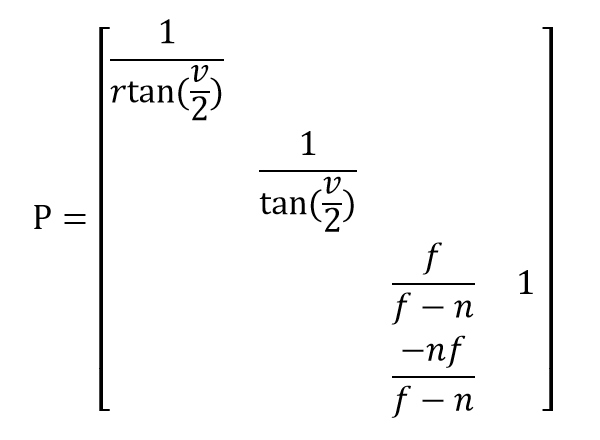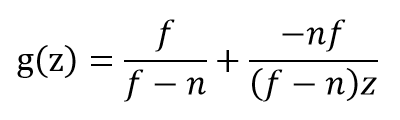``````% gen_gz.m

n = [  1,  1,   1 ];
f = [ 10, 20, 100 ];

z = 0:0.01:max(f);

% size(..) returns [col, row]
size_n = size(n); size_f = size(f);
group = min([size_n(2), size_f(2)]);

info = cell(1, group);
for index = 1:group
crnt_n = n(index);
crnt_f = f(index);
temp_a = crnt_f / (crnt_f - crnt_n);
temp_b = - (crnt_n * crnt_f) ./ ((crnt_f - crnt_n) * z);
g = temp_a + temp_b;
% draw curves in a figure
info{index} = ['g(z)', ',n=', num2str(crnt_n), ',f=', num2str(crnt_f)];
plot(z, g, 'LineWidth', 1.2);
hold on
end
axis([0 max(f) 0 1]);
legend(info);
grid on

clear crnt_n crnt_f temp_a temp_b index g
``````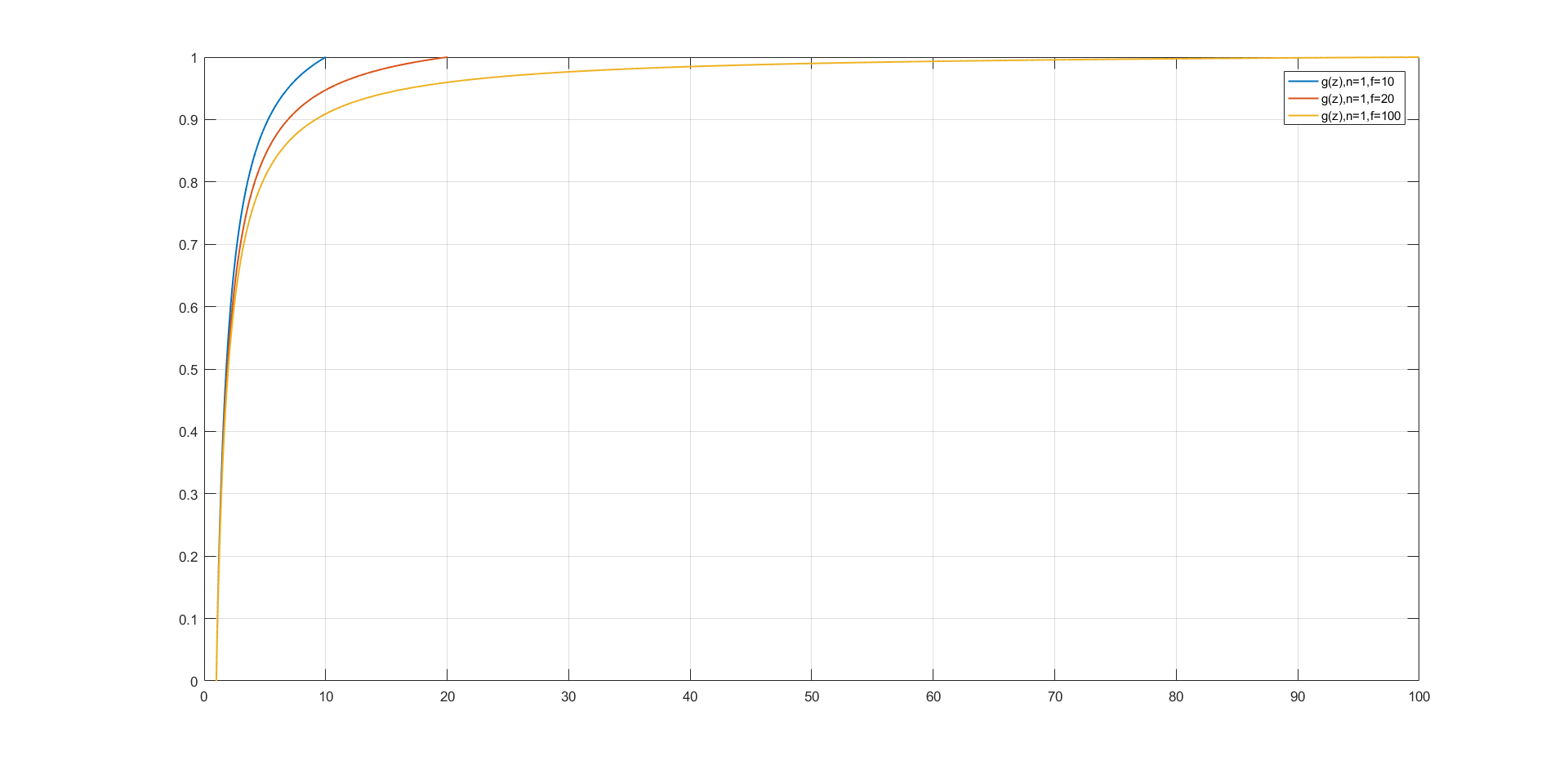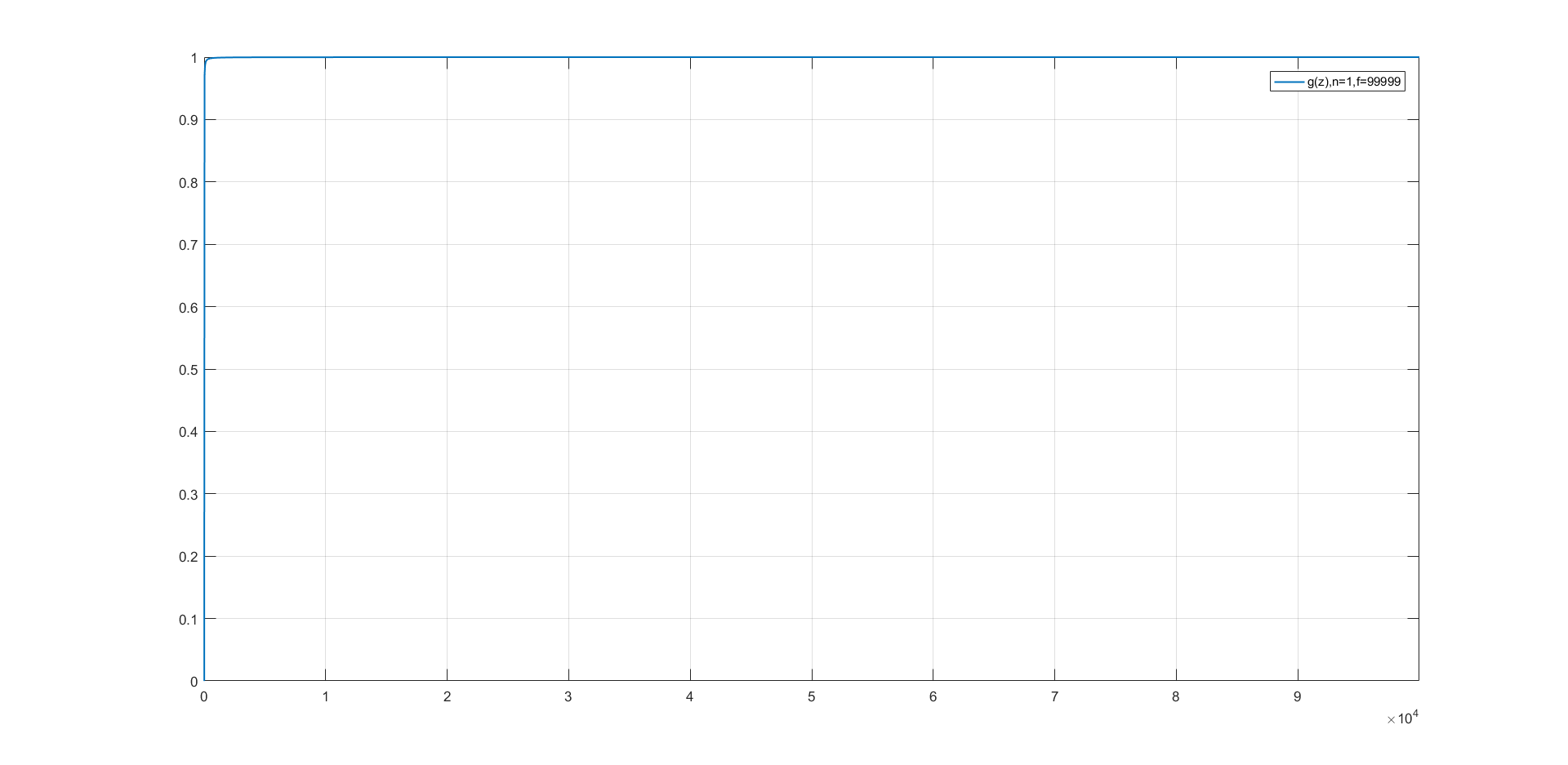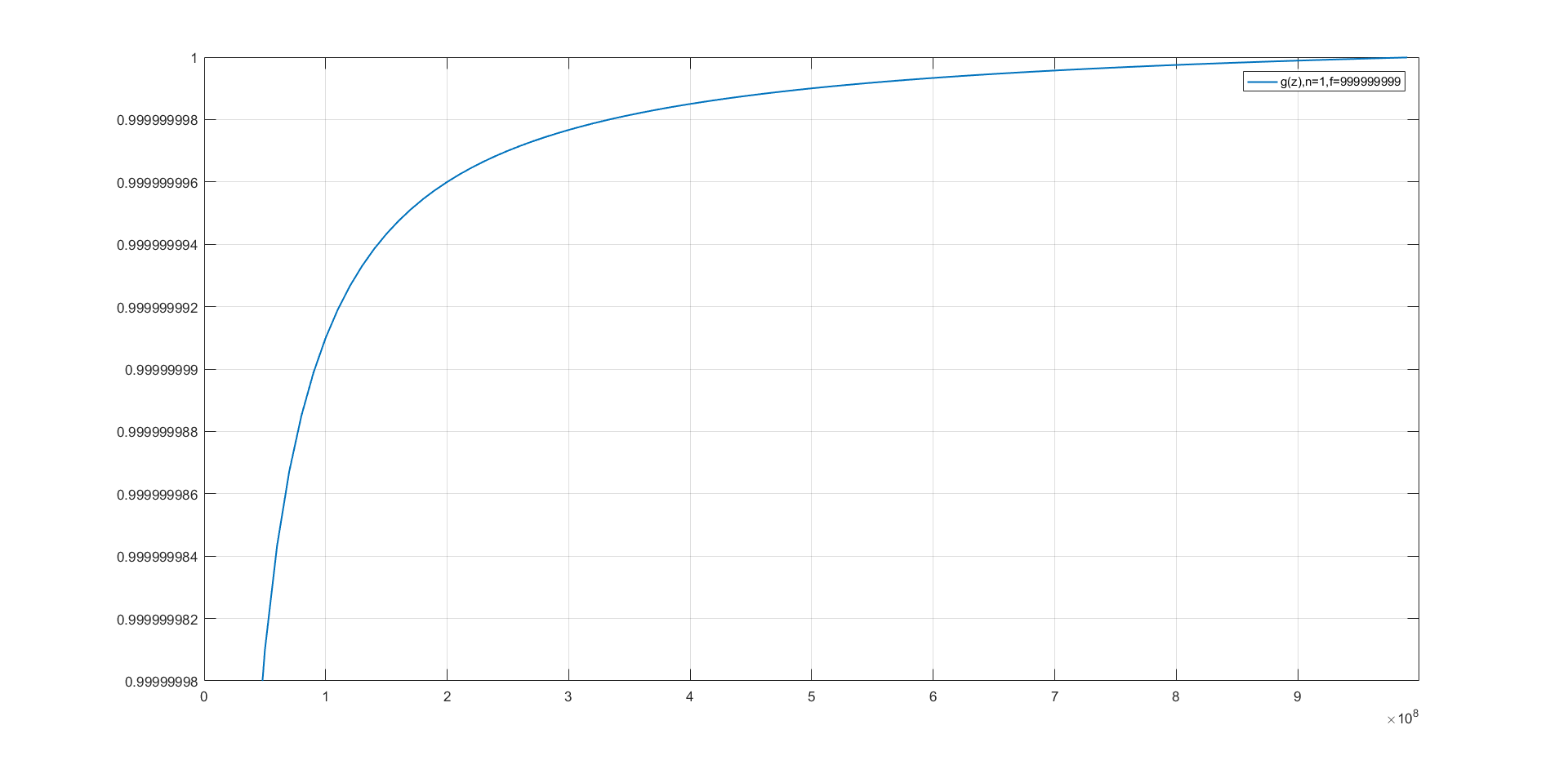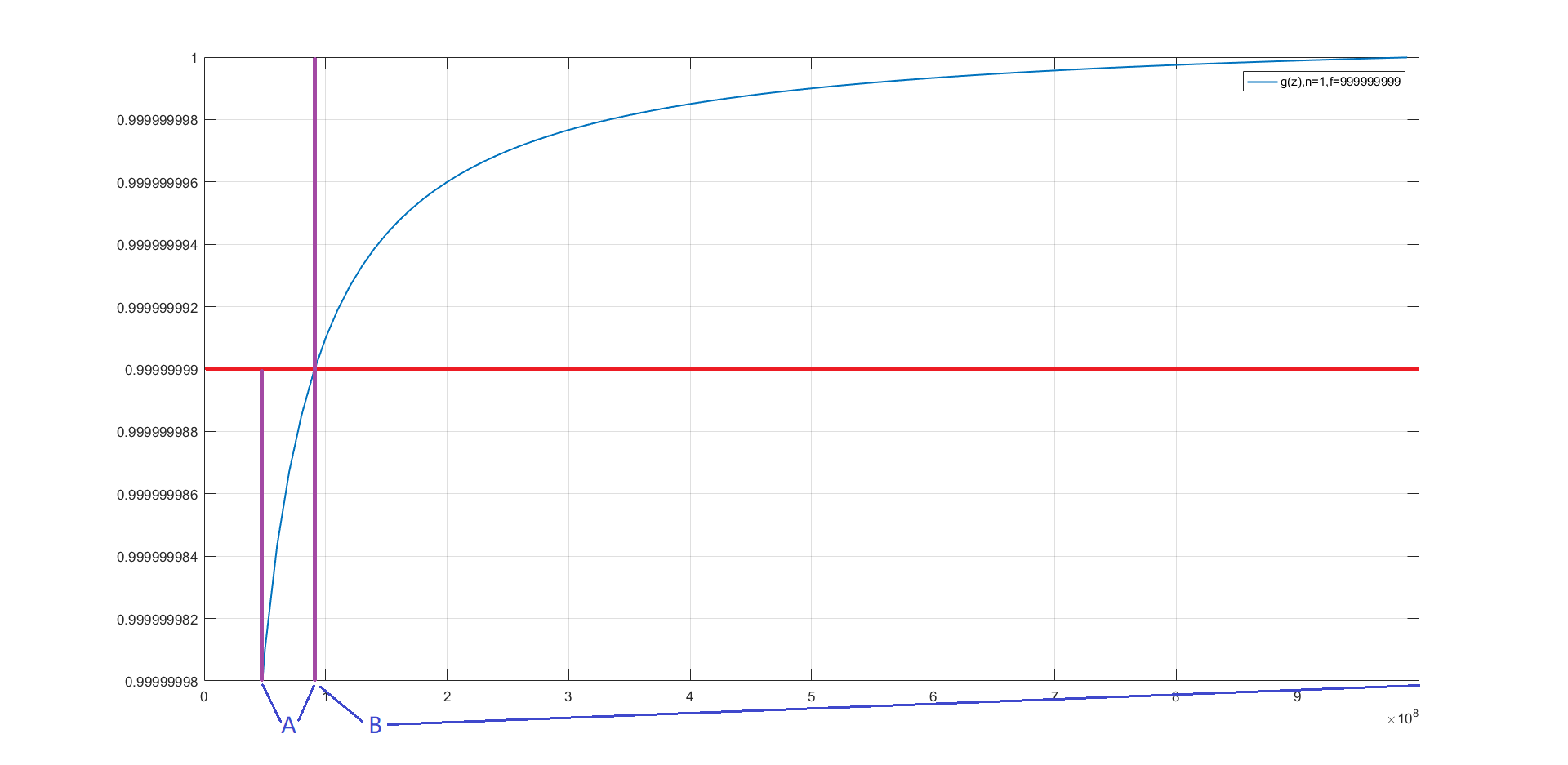×